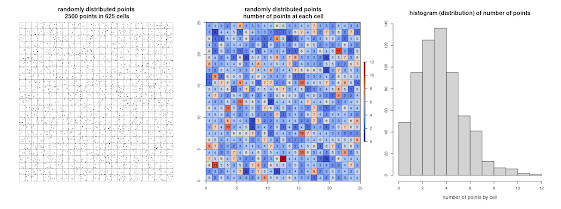## viernes, 22 de enero de 2021

### Are betting houses randomly distributed in Madrid? Point pattern analysis in R

Hi there!

Last December 13th there was a demonstration in Carabanchel, my home neighborhood in Madrid (Spain) against the proliferation of betting houses (small casinos where you can get cheap drinks or coffees while you spend your money betting or gambling). Many neighborhood associations usually complain against this kind of places arguing they bait young people with very low prices, who can develop gambling addiction in the future. Moreover some organizations ensure that the proliferation of this kind of places is focused in working class neighborhoods with low incomes and high unemployed rates (see this great report for more information about that - in Spanish). So, how could we know if betting houses are randomly distributed in Madrid?

Imagine that you have a 25x25 (625 cells) square grid and you want to scatter 2500 points. If the points are randomly distributed, they will have the same probability of occupy whatever cell. That is, the first point will have a probability of 1/625 of being in cell number 1, the same probability (1/625) probability of being at cell number 2, and so on. Thus, if we have 2500 points in 625 cells, each cell would have 2500/625 = 4 points in average. This is the meaning of the lambda from a Poisson distribution, the mean or the most probable value.Imagine now that we have the same points in the same grid, but now they are clustered in 3 groups. In this dummy example, we can easily guess that they are not randomly distributed by just observing the point pattern. But how could we demonstrate mathematically/numerically they are not randomly distributed?

Well, given we know the shape of the histogram (distribution) that should have a completely random distribution (in our case a Poisson(λ=4)), an easy and straightforward way to demonstrate mathematically that the second point patter is not random at all would be to compare both distributions using for example a Kolmogorov–Smirnov test in R.

Lets do the same with betting houses in Madrid city (Spain). Betting places locations can be downloaded from the city government website, as well as other data such as neighborhood limits or family income per neighborhood.I prepared a .zip with the needed files which can be downloaded from here.

There are currently 409 betting houses. We will divide the city in a grid of 18x18 cells of 1 km size as follows

`````` # Load required packages
library(rgdal)
library(raster)
library(dismo)
library(GISTools)

# Create the grid
bh.sp <- SpatialPointsDataFrame(coords=bh[,1:2], data=bh)
ext <- extent(c(min(bh\$x),min(bh\$x)+18000,min(bh\$y),min(bh\$y)+18000))
landscape <- raster(ncols=18,nrows=18,ext=ext, vals=1:324)
grid <- rasterToPolygons(landscape)

# Plot betting houses in Madrid
par(mar=c(0.1,0.1,1,0.1))
plot(nb, main = "City of Madrid")
plot(grid, lwd = 0.4, add = TRUE)
points(bh.sp, pch=16, cex=0.7, col="black")
``````

Cool. Since Madrid is an heterogeneous city, we will select for our analysis only those cells in which there is one or more betting houses. Then, we'll spread random points in the selected squares to compare both histograms/distributions: random points VS betting houses.

`````` # Select cells with betting houses
grid\$bh <- poly.counts(bh.sp, grid)
sel.sqr <- (grid[grid\$bh > 0,])
landscape[grid\$bh == 0] <- NA

# Plot
par(mar=c(0.1,0.1,0.1,0.1))
plot(sel.sqr)
lines(nb, lwd = 0.2)
points(bh.sp, pch=16, cex=0.7, col="black")

# Add random points in selected cells
rp <- randomPoints(disaggregate(landscape, 100), nrow(bh))
points(rp, pch=16, cex=0.7, col = "red" )
rp.sp <- SpatialPointsDataFrame(coords=rp[,1:2], data=data.frame(rp))
``````

Well done! We have selected 118 cells with at least one betting house and we have scattered 409 random points on them. So, now the last task is to count the number of betting houses and the number of random points at each cell. Then, we could compare both histograms/ distributions, and decide if they present a similar pattern.

`````` par(mfrow = c(1,2))
hist(poly.counts(rp.sp, sel.sqr), right=F, main = "random points",
xlab="number of random points by cell")
hist(poly.counts(bh.sp, sel.sqr), right=F, main = "betting houses",
xlab="number of betting houses by cell")

``````

As you can see, under a random point pattern the most frequent value is between 3 and 4. Since we have 409 points randomly distributed over 108 cells, we expected to have 409/108 = 3.78 points at each cell. It looks pretty good!

However, we can see that the most frequent value in the betting house histogram is between 0 and 2... This is because there are many empty cells, while there are some of them with high number of betting houses (a clustered pattern). We could compare both distributions mathematically using a Kolmogorov–Smirnov test.

`````` #Kolmogorov–Smirnov test
ks.test(poly.counts(rp.sp, sel.sqr), poly.counts(bh.sp, sel.sqr))
``````

Bonus Track: so, what variable or variables drive the distribution of betting houses in Madrid? Here you have a clue... but take the data and explore it yourself!

Stay safe!